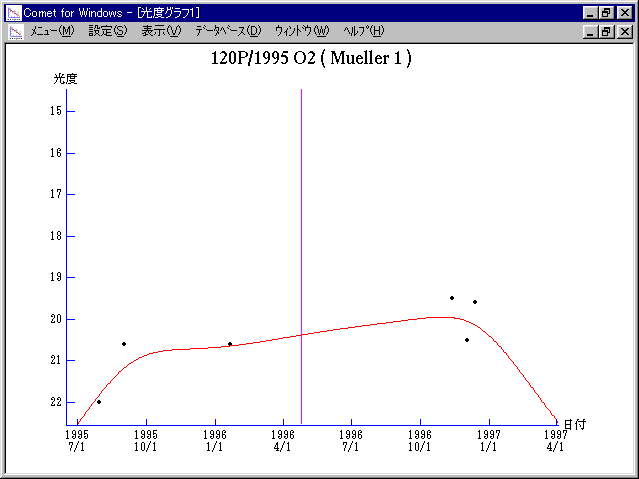# \$B%_%e%i!

120P/Mueller 1 (1996)###\$B%W%m%U%#!<%k(B

 \$BId9f(B 120P/1995 O2 \$B8!=PF|(B 1995\$BG/(B7\$B7n(B30\$BF|(B \$B8!=P8wEY(B 22.2\$BEy(B \$B8!=P J. V. Scotti (Kitt Peak)

###\$B50F;MWAG(B

```   The following improved orbital elements by Kenji Muraoka, are
from 57 observations 1987 to 1996, perturbations by 9 Plantes,
Moon and 5 minor planets were taken into account.
The mean residual is +/- 0.79 arc seconds.

Epoch  =  1996 Apr. 27.0  TT       JDT = 2450200.5
T  =  1996 Apr. 24.64954       +/- 0.00388 (m.e.) TT
Peri. =   29.91671                +/- 0.00098
Node  =    4.56183                +/- 0.00018   (2000.0)
Incl. =    8.79596                +/- 0.00004
q  =    2.7394934              +/- 0.0000012 AU
e  =    0.3373750              +/- 0.0000003
a  =    4.1343044              +/- 0.0000007 AU
n  =    0.11724664             +/- 0.00000003
P  =    8.406                  +/- 0.0000021  years
```

###\$B@1?^(B1995\$BG/(B 6\$B7n(B 2\$BF|!A(B1997\$BG/(B 2\$B7n(B21\$BF|(B

###\$B8wEYJQ2=(B

```        m1 = 3.0 + 5 log\$B&\$(B + 33 log r(t - 45)
```##### \$B50F;MWAG\$OB<2,7r<#;a\$N7W;;\$K\$h\$k\$b\$N\$G\$9!#(B \$B@1?^\$O(B StellaNavigator Ver.2.0 for Windows (\$B%"%9%H%m%"!<%D(B \$BJTCx(B / \$B%"%9%-!<=PHG6I4)(B) \$B\$G:n@.\$7\$?\$b\$N\$G\$9!#(B \$B8wEY%0%i%U\$O(BComet for Windows\$B\$G:n@.\$7\$?\$b\$N\$G\$9!#(B